BP神经网络：

BP神经网络计算原理：

BP网络的MATLAB实践

【举例】对汽油辛烷值含量做预测：

# 什么是人工神经网络（ANN）?

In machine learning and cognitive science, artificial neural networks (ANNs) are a family of statistical learning models inspired by biological neural networks (the central nervous systems of animals, in particular the brain) and are used to estimate or approximate functions that can depend on a large number of inputs and are generally unknown.

# 人工神经元模型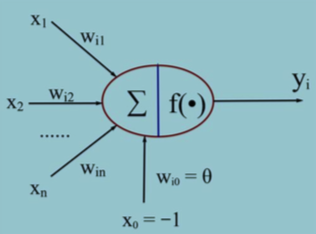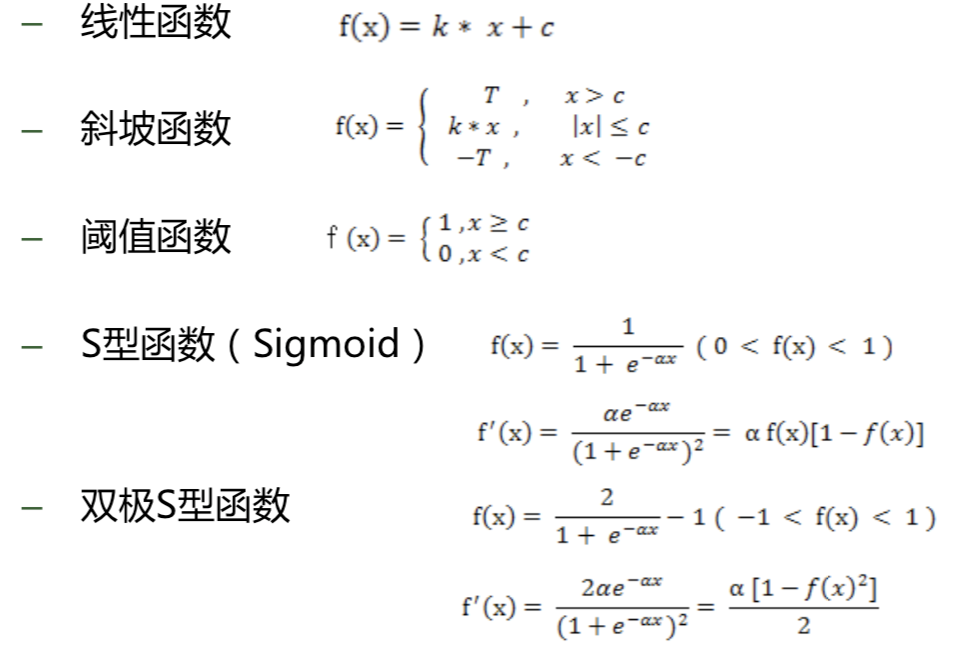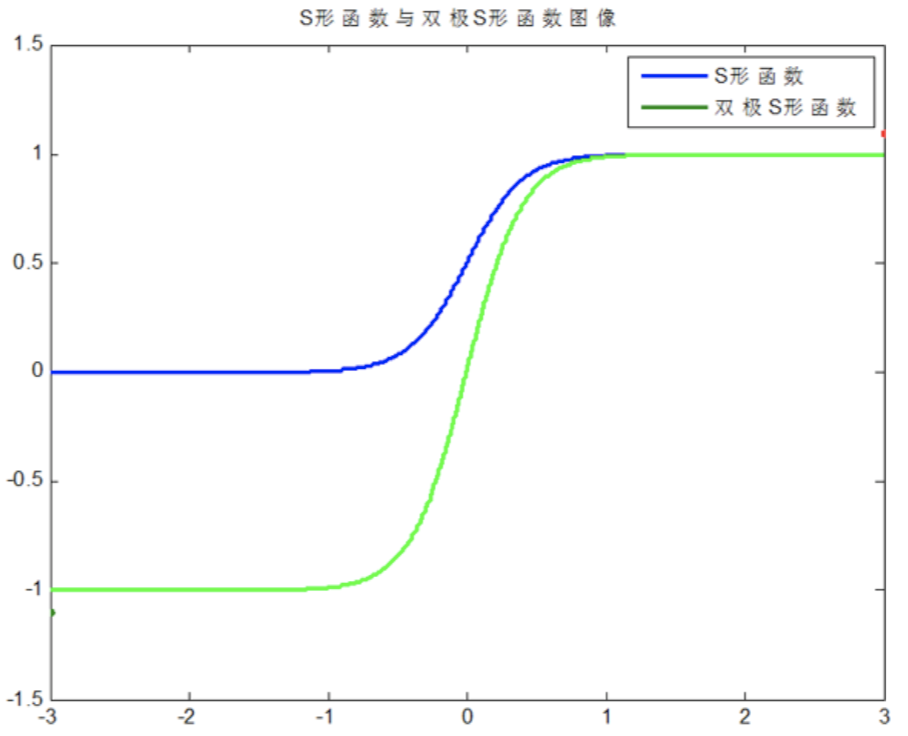• –  按照连接方式，可以分为:前向神经网络 vs. 反馈(递归)神经网络

• –  按照学习方式，可以分为:有导师学习神经网络 vs. 无导师学习神经网络

• –  按照实现功能，可以分为:拟合(回归)神经网络 vs. 分类神经网络

# BP神经网络：

1. Forward propagation of a training pattern’s input through the neural network in order to generate the propagation’s output activations.

2. Back propagation of the propagation’s output activations through the neural network using the training pattern’s target in order to generate the deltas of all output and hidden neurons.

1. Multiply its output delta and input activation to get the gradient of the weight.

2. Bring the weight in the opposite direction of the gradient by subtracting a ration of it from the weight.

## BP神经网络计算原理：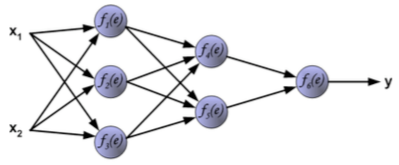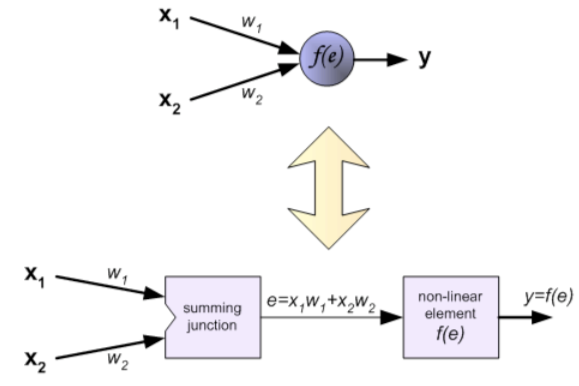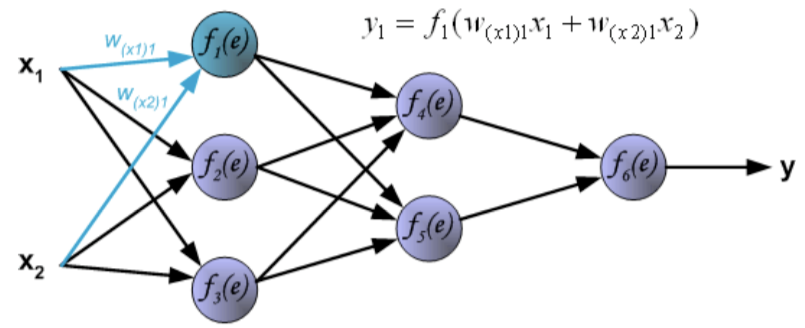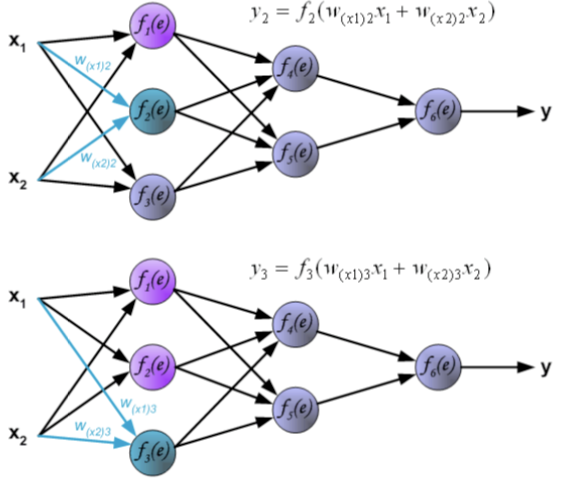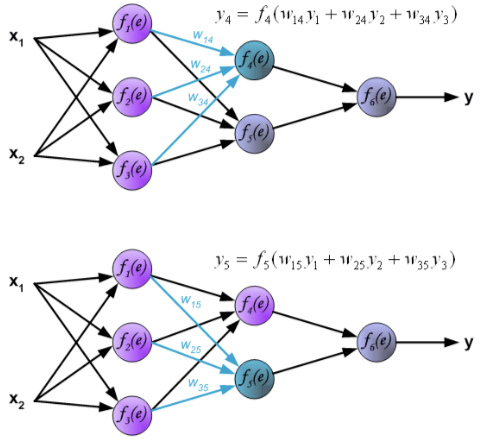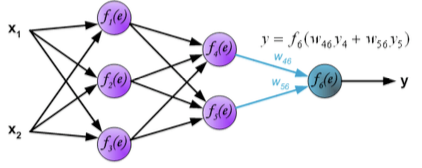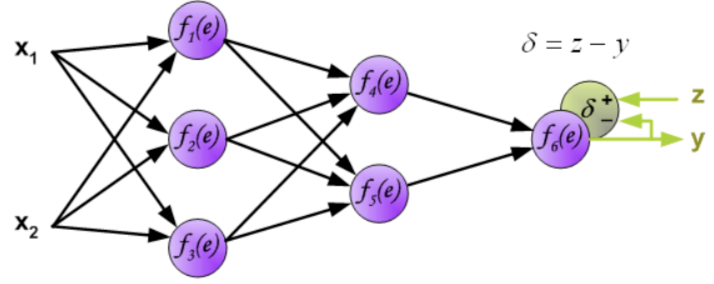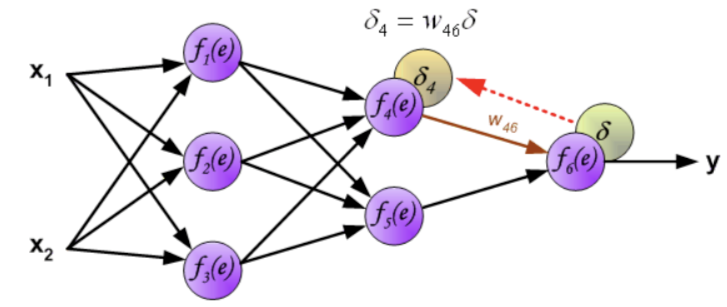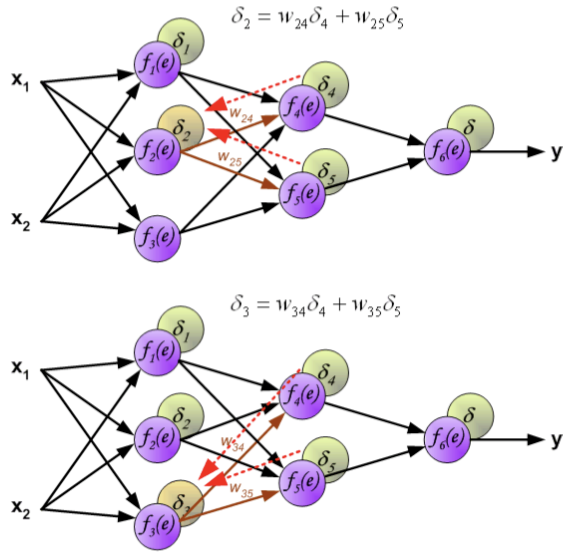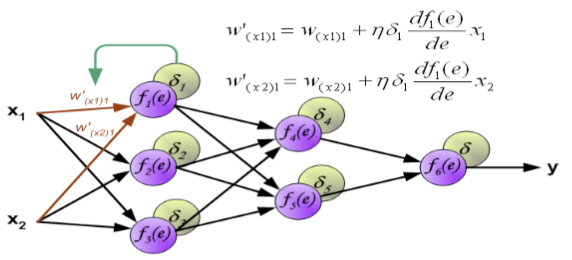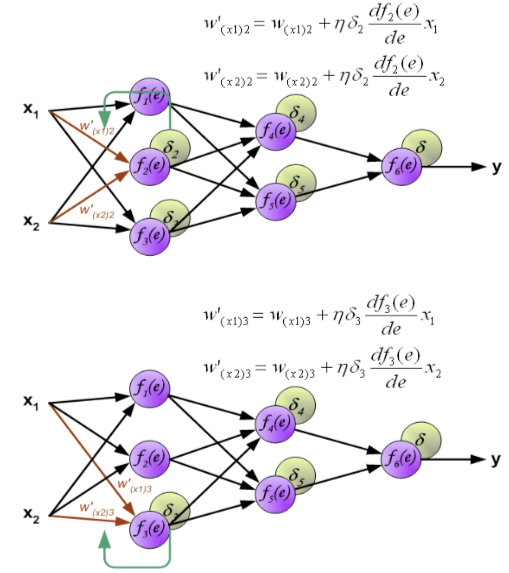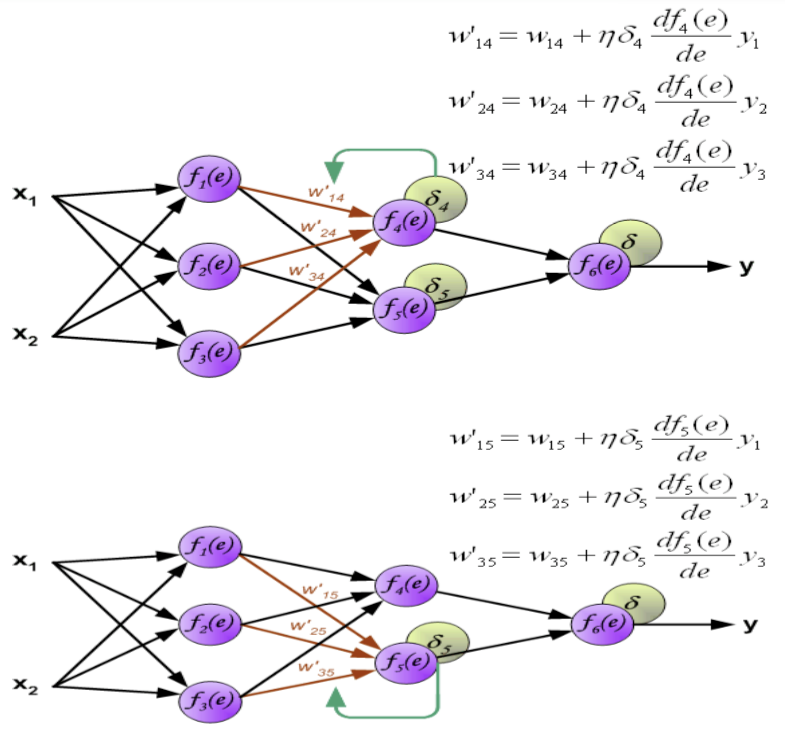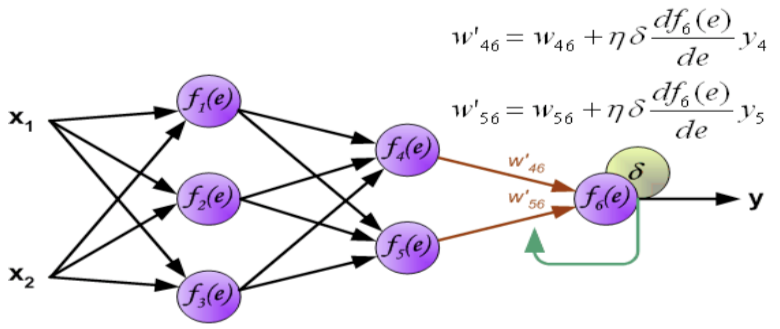## 归一化

——将数据映射到[0, 1]或[-1, 1]区间或其他的区间。

• –  输入数据的单位不一样，有些数据的范围可能特别大，导致的结果是神经网络收敛慢、训练时间长。

• –  数据范围大的输入在模式分类中的作用可能会偏大，而数据范围小的输入作用就可能会偏小。(防止数据湮灭等现象)

• –  由于神经网络输出层的激活函数的值域是有限制的，因此需要将网络训练的目标数据映射到激活函数的值域。例如神经网络的输出层若采用S形激活 函数，由于S形函数的值域限制在(0,1)，也就是说神经网络的输出只能限制在(0,1)，所以训练数据的输出就要归一化到[0,1]区间。

• –  S形激活函数在(0,1)区间以外区域很平缓，区分度太小。例如S形函数f(X)在参数a=1时，f(100)与f(5)只相差0.0067。这样数据的差异就会失去意义！

• y = ( x - min )/( max - min )  —— 【0,1】

• –  y = 2 * ( x - min ) / ( max - min ) - 1 —— 【-1,1】

## BP网络的MATLAB实践

• mapminmax

• –  Process matrices by mapping row minimum and maximum values to [-1 1]

• –  [Y, PS] = mapminmax(X, YMIN, YMAX)  归一化的信息保存到结构体PS中（自定义MIN,MAX范围）

• –  Y = mapminmax('apply', X, PS)  已知的归一化规则PS用于归一化其他信息

• –  X = mapminmax('reverse', Y, PS)  反归一化

• newff

• –  Create feed-forward backpropagation network  创建前向型神经网络

• –  net = newff(P, T, [S1 S2...S(N-l)], {TF1 TF2...TFNl}, BTF, BLF, PF, IPF, OPF, DDF)  输入样本、输出样本、传递函数……等其他参数。

• train

• –  Train neural network  训练神经网络

• –  [net, tr, Y, E, Pf, Af] = train(net, P, T, Pi, Ai)

• sim

• – Simulate neural network 模拟预测

• – [Y, Pf, Af, E, perf] = sim(net, P, Pi, Ai, T)  Y即最终预测的输出

## 参数对BP神经网络性能的影响：

1、隐含层神经元节点个数 ？

2、激活函数类型的选择 ？

newff里会默认设计好（tansig，双S型），还可以是其他函数。

3、学习率？——参考网络资料。

4、初始权值与阈值:

1、交叉验证(cross validation)：训练集(training set)+验证集(validation set)+测试集(testing set)。保证足够的样本，可以分到这三波里。
2、留一法(Leave one out, LOO)：如果样本数据较少时使用。每一次都留一个样本做预测，其他用于训练。

## 【举例】对汽油辛烷值含量做预测：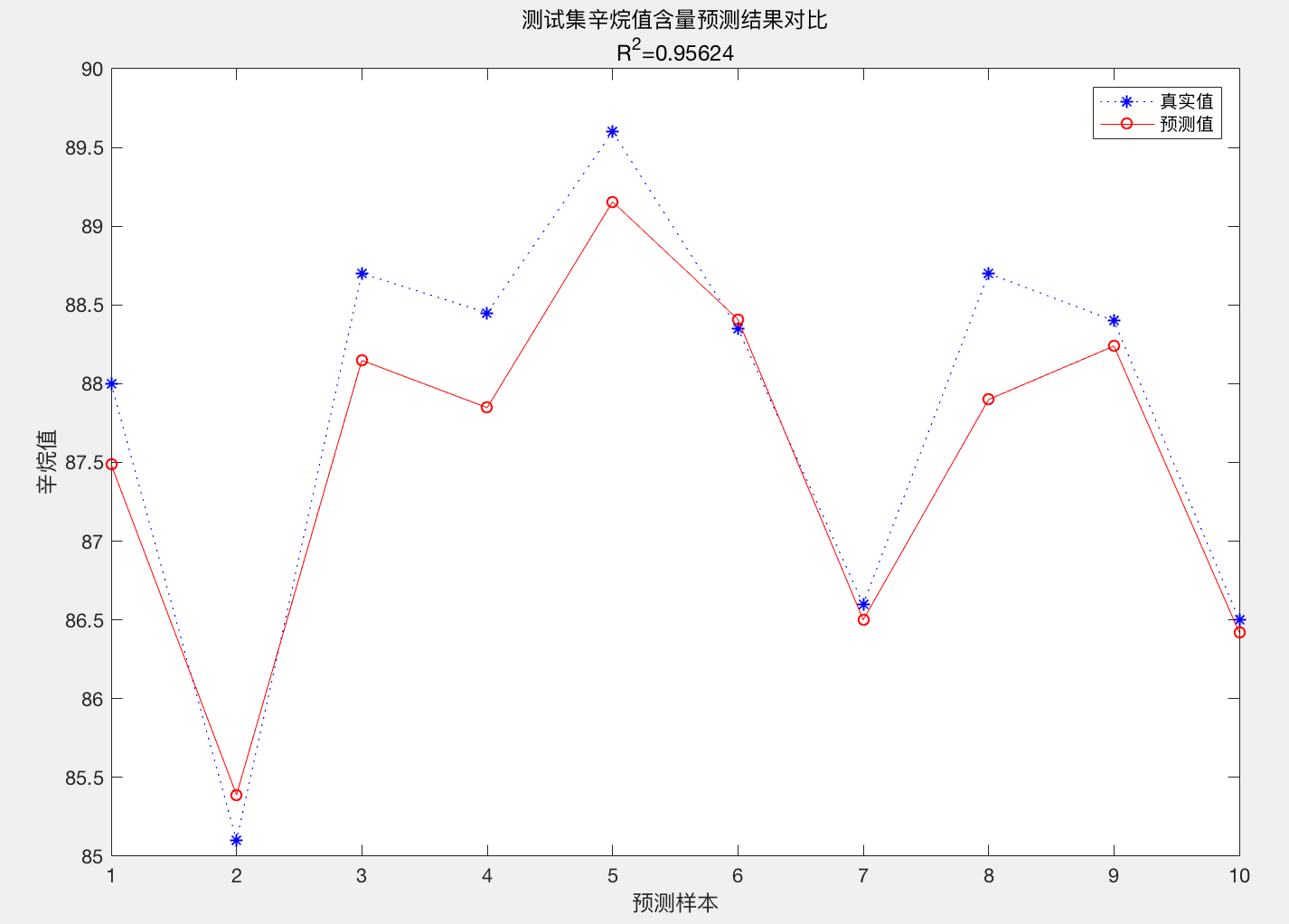matlab代码：



%
% I. 清空环境变量

clear all

clc

%
% II. 训练集/测试集产生

%
%

%
1. 导入数据

%
%

%
2. 随机产生训练集和测试集

temp = randperm(size(NIR,1));

%
训练集――50个样本

P_train = NIR(temp(1:50),:)';

T_train = octane(temp(1:50),:)';

%
测试集――10个样本

P_test = NIR(temp(51:end),:)';

T_test = octane(temp(51:end),:)';

N = size(P_test,2);

%
% III. 数据归一化

[p_train, ps_input] = mapminmax(P_train,0,1);

p_test = mapminmax('apply',P_test,ps_input);

[t_train, ps_output] = mapminmax(T_train,0,1);

%
% IV. BP神经网络创建、训练及仿真测试

%
%

%
1. 创建网络

net = newff(p_train,t_train,9);

%
%

%
2. 设置训练参数

net.trainParam.epochs = 1000;

net.trainParam.goal = 1e-3;

net.trainParam.lr = 0.01;

%
%

%
3. 训练网络

net = train(net,p_train,t_train);

%
%

%
4. 仿真测试

t_sim = sim(net,p_test);

%
%

%
5. 数据反归一化

T_sim = mapminmax('reverse',t_sim,ps_output);

%
% V. 性能评价

%
%

%
1. 相对误差error

error = abs(T_sim - T_test)./T_test;

%
%

%
2. 决定系数R^2

R2 = (N * sum(T_sim .* T_test) - sum(T_sim) * sum(T_test))^2 / ((N * sum((T_sim).^2) - (sum(T_sim))^2) * (N * sum((T_test).^2) - (sum(T_test))^2));

%
%

%
3. 结果对比

result = [T_test' T_sim' error']

%
% VI. 绘图

figure

plot(1:N,T_test,'b:*',1:N,T_sim,'r-o')

legend('真实值','预测值')

xlabel('预测样本')

ylabel('辛烷值')

string = {'测试集辛烷值含量预测结果对比';['R^2=' num2str(R2)]};

title(string)



http://www.cnblogs.com/xfzhang/archive/2013/05/24/3096412.html

2、matlab_geeker，http://edu.dataguru.cn

3、维基百科

07-1403-27
05-066万+
08-25
12-013万+
05-282万+